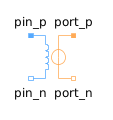Standard Deviation - MapleSim Help

Standard Deviation

Calculates the empirical standard deviation of its input signalDescription The Standard Deviation component calculates the standard deviation of its input signal. The standard deviation is the square root of the signal's variance. The Variance block is used to calculate the variance. The parameter ${t}_{\mathrm{\epsilon }}$ is used to guard against division by zero (the computation starts at ${t}_{0}+{t}_{\mathrm{\epsilon }}$ and before that time instant $y=0$).Connections

 Name Description Modelica ID $u$ Noisy input signal u $y$ Standard deviation of the input signal yParameters

 Name Default Units Description Modelica ID ${t}_{\mathrm{\epsilon }}$ $1·{10}^{-7}$ $s$ Standard deviation calculation starts at ${t}_{0}+{t}_{\mathrm{\epsilon }}$ t_epsModelica Standard Library The component described in this topic is from the Modelica Standard Library. To view the original documentation, which includes author and copyright information, click here.